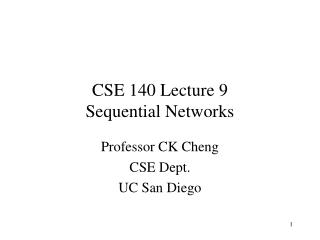# CSE 140 Lecture 9 Sequential Networks - PowerPoint PPT PresentationDownload PresentationCSE 140 Lecture 9 Sequential Networks

Presentation Description
Download Presentation## CSE 140 Lecture 9 Sequential Networks

- - - - - - - - - - - - - - - - - - - - - - - - - - - E N D - - - - - - - - - - - - - - - - - - - - - - - - - - -
##### Presentation Transcript

1. CSE 140 Lecture 9Sequential Networks Professor CK Cheng CSE Dept. UC San Diego

2. Sequential Networks Combinational D B C A CLK CLK • Components F-Fs • Specification • Implementation: Excitation Table

3. Specification • Finite State Machine: • Input Output Relation • State Diagram • State Table • Circuit: • Logic Diagram • Netlist • Boolean Expression

4. x D1 Q1 Q0 Q D Q’ Q0 y Q D Q1 Q’ D0 Clk Netlist  State Table  State Diagram Input Output Relation y(t) = Q1(t)Q0(t) Q0(t+1) = D0(t) = x(t)Q1(t) Q1(t+1) = D1(t) = x(t) + Q0(t)

5. Netlist  State Table  State Diagram Input Output Relation x D1 Q1 Q0 Q D Q’ y Q D Q0 Q1 Q’ D0 Clk y(t) = Q1(t)Q0(t) Q0(t+1) = D0(t) = x(t)Q1(t) Q1(t+1) = D1(t) = x(t) + Q0(t)

6. input x=0 x=1 PS S0 S1 S2 S3 S0, 0 S2, 0 S2, 0 S2, 0 S0, 0 S3, 0 S2, 1 S3, 1 Logic Diagram => State Table y(t) = Q1(t)Q0(t) Q0(t+1) = D0(t) = x(t) Q1(t) Q1(t+1) = D1(t) = x(t) + Q0(t) State table input Let: S0 = 00 S1 = 01 S2 = 10 S3 = 11 x=0 x=1 PS 00 01 10 11 00, 0 10, 0 10, 0 10, 0 00, 0 11, 0 10, 1 11, 1 Q1(t)Q0(t)Q1(t+1)Q0(t+1), y(t) Remake the state table using symbols instead of binary code , e.g. ’00’

7. x/y 1/1 0/0 0/0 1/0 0,1/0 S2 S3 S1 S0 0/1 1/0 input x=0 x=1 PS S0 S1 S2 S3 S0, 0 S2, 0 S2, 0 S2, 0 S0, 0 S3, 0 S2, 1 S3, 1 State Table => State Diagram Example: Output sequence

8. X T0 Q0 Q T Q’ y Q Q1 T Q’ T1 Example with T Flip-Flops y(t) = Q1(t)Q0(t) Q0(t+1) = T0(t) = x(t) Q1(t) Q1(t+1) = T1(t) = x(t) + Q0(t)

9. Logic Diagram => Excitation Table => State Table y(t) = Q1(t)Q0(t) T0(t) = x(t) Q1(t) T1(t) = x(t) + Q0(t) Q0(t+1) = T0(t) Q’0(t)+T’0(t)Q0(t) Q1(t+1) = T1(t) Q’1(t)+T’1(t)Q1(t) Excitation Table

10. Excitation Table =>State Table => State Diagram State Assignment S0 00 S1 01 S2 10 S3 11 1/1 0/0 0/1 S0 S1 S3 1/0 0, 1/0 1/0 S2 0/0

11. 1/1 0/0 0/1 S0 S1 S3 1/0 0, 1/0 1/0 S2 0/0 Excitation Table =>State Table => State Diagram Example: Output sequence

12. Implementation: State Diagram => State Table => Netlist Pattern Recognizer: A sequential machine has a binary input x in {a,b}. For x(t-2, t) = aab, the output y(t) = 1, otherwise y(t) = 0. b/1 b/0 S1 S0 a/0 a/0 S2 a/0 b/0

13. State Diagram => State Table with State Assignment State Assignment S0: 00 S1: 01 S2: 10 Q1(t+1)Q0(t+1), y a: 0 b: 1

14. State Table => Excitation Table

15. Q0 D1(t): 0 2 6 4 0 1 - 1 1 3 7 5 0 0 - 0 x(t) Q1 Excitation Table => Boolean Expression D1(t) = x’Q0 + x’Q1 D0 (t)= Q’1Q’0 x’ y= Q1x

16. Q’1 Q0 D0 Q Q’0 D x’ Q’ Q1 y x’ D1 Q D Q0 Q’ Q1 x D1(t) = x’Q0 + x’Q1 D0 (t)= Q’1Q’0 x’ y= Q1x

17. Canonical Form: Mealy and Moore Machines x(t) y(t) Combinational Logic CLK x(t) C2 y(t) x(t) C1 C2 y(t) C1 CLK CLK

18. Canonical Form: Mealy and Moore Machines Mealy Machine: yi(t) = fi(X(t), S(t)) Moore Machine: yi(t) = fi(S(t)) si(t+1) = gi(X(t), S(t)) x(t) x(t) C1 C2 y(t) C1 C2 y(t) CLK CLK s(t) s(t) Moore Machine Mealy Machine

19. Finite State Machine Example • Traffic light controller • Traffic sensors: TA, TB (TRUE when there’s traffic) • Lights: LA, LB

20. FSM Black Box • Inputs: CLK, Reset, TA, TB • Outputs: LA, LB

21. FSM State Transition Diagram • Moore FSM: outputs labeled in each state • States: Circles • Transitions: Arcs

22. FSM State Transition Diagram • Moore FSM: outputs labeled in each state • States: Circles • Transitions: Arcs

23. FSM State Transition Table

24. State Transition Table Q1(t+1)= Q1(t)Å Q0(t) Q0(t+1)= Q’1(t)Q’0(t)T’A + Q1(t)Q’0(t)T’B

25. FSM Output Table LA1 = Q1 LA0 = Q’1Q0 LB1 = Q’1 LB0 = Q1Q0

26. FSM Schematic: State Register

27. Logic Diagram

28. FSM Schematic: Output Logic